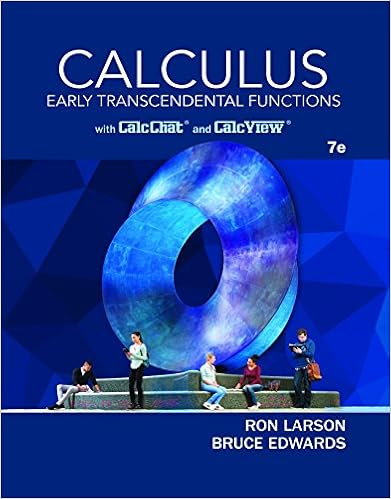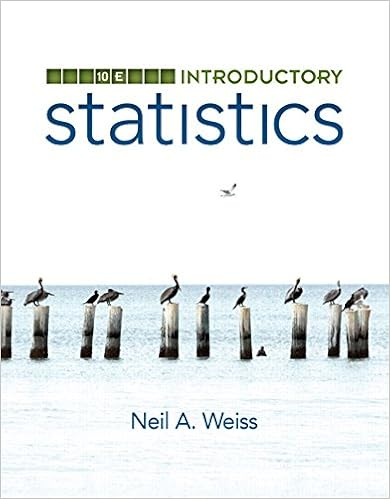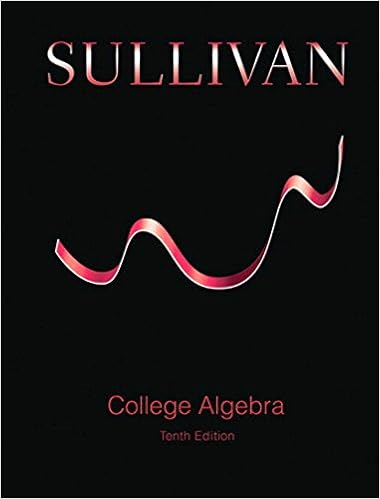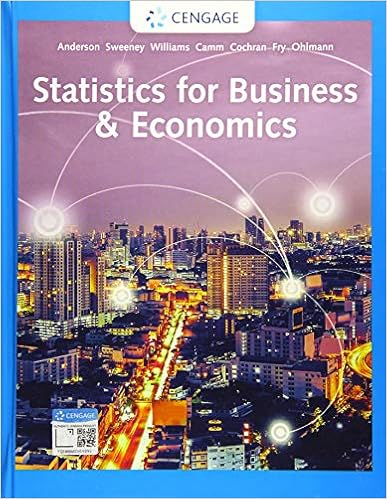# 7 the product of two positive numbers is 100 what

• Homework Help
• 18
• 95% (19) 18 out of 19 people found this document helpful

This preview shows page 9 - 15 out of 18 pages.

7.The product of two positive numbers is 100. What numbers will produce the leastpossible sum? Confirm that the sum is in fact a minimum. (8 marks)2
##### We have textbook solutions for you!The document you are viewing contains questions related to this textbook.
Chapter 11 / Exercise 02
Calculus: Early Transcendental Functions
Edwards/LarsonExpert VerifiedBrowse all Textbook Solutions
x=100x=10y=10010y=10s=x+ys=10+10s=20d2dx2=x+100xf '(x)=1100x2f ' '(x)=200x3f' '(10)=200(10)3f' '(10)=15=0.20Therefore, the minimum value is 20.
8.ABCis a triangle whereAis(1,3),Bis(−2,1), and(−1,4). Determine whetherABCis a right-angle triangle.Cis
Task #3: Communication Questions9.Discuss how displacement, velocity, and acceleration are related to a function and itsderivatives. Create an equation for the displacement of an object and determine theequations for the velocity and acceleration of the object at any timet. (6 marks)
t=0.59sEquation for Velocity:v(t)=s '(t)v(t)=0.12(t)v(0.59)=0.12(0.59)v(0.59)=0.071m/sEquation for Acceleration:a(t)=v'(t)a(t)=0.24m/s210. What is the difference between a scalar and a vector quantity? Include an example ofeach. (4 marks)
Scalar Quantity Example: AreaVector Quantity Example: Acceleration11. List and describe the three properties of vector arithmetic. Give an example of eachproperty using vectors in Cartesian form. (12 marks: 4 marks for each property))
¿(−1,13)u+(v+w)=(2,3)+[(1,5)+(2,5)]12¿(5+5,(¿)]¿(2,3)+¿¿(2,3)+(3,10)¿(−1,13)Therefore,(u+v)+w=u+(v+w).2.Distributive Property of Scalar MultiplicationFor any scalar quantity a and vector quantitiesuv:a(u+v)=au+avoExample:a(u+v)= 5[(4, 3,7) + (-3, 0, 6)]= [(20, 15, 35) + (-15, 0, 30)]= (5, 15, 65)au+av= 5(4, 3, 7) + 5(-3, 0, 6)= (20, 15,35) + (-15, 0, 30)= (5, 15, 65)Therefore,a(u+v)=au+av.3.Associative Property of Vector Addition
For any three vectorsu,vw:(u+v)+w=u+(v+w)oExample:(u+v)+w=[(3,5)+(6,4)]+(2,0)¿[(36),(5+4

Course Hero member to access this document

Course Hero member to access this document

End of preview. Want to read all 18 pages?

Course Hero member to access this document

Term
Summer
Professor
N/A
Tags
Velocity, Vector Motors
##### We have textbook solutions for you!
The document you are viewing contains questions related to this textbook.The document you are viewing contains questions related to this textbook.
Chapter 11 / Exercise 02
Calculus: Early Transcendental Functions
Edwards/LarsonExpert Verified
•••